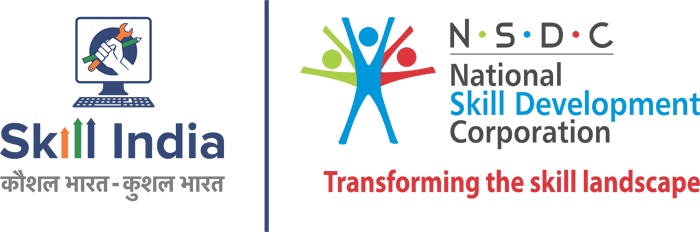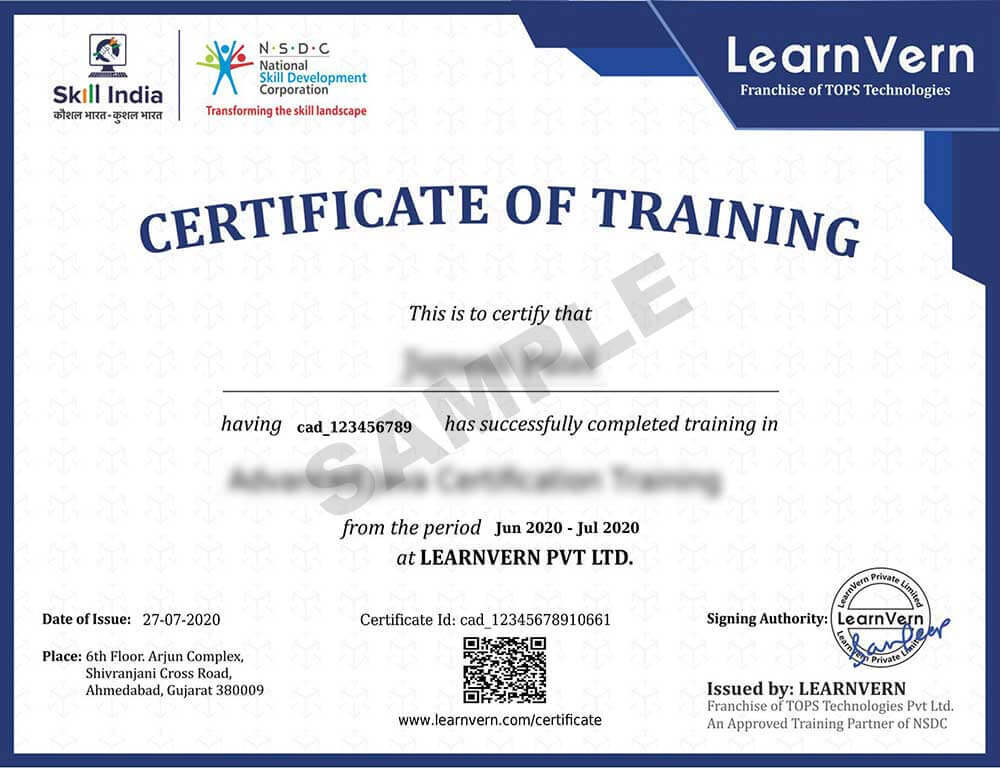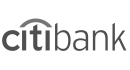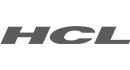# Matlab Programming Course

Course Rating

4.7 (74)

Active Learners#### What’s included in this Course11+ hours of on-demand video31 Articles7 Exercise25 Downloadable ResourcesAccess on Mobile and TVLife Time Access

Authorized Training Partner of### Overview

Want to learn MATLAB? Learn MATLAB fundamentals and MATLAB programming language. We have the best MATLAB tutorial videos for free. Our tutorials come with examples and exercises so you can practice too. Free MATLAB Course. Enroll now.

LearnVern’s MATLAB course by Industry Experts is a thoroughly free online course that offers you knowledge about data analysis, complex numbers, logical operators, linear algebra, matrix, the help command, linear equations, the plot function, inverse matrix, relational operators, the use of the semicolon, the eigenvalues, while statement, if statement, two-dimensional mesh grid, data types; and all the concepts and tools necessary for you to become a Matlab programmer. We offer MATLAB coding, MATLAB simulink tutorial, MATLAB basics tutorial, MATLAB video, and more. Get started today!

Discover the best way to learn MATLAB with our MATLAB training course, developed by subject matter experts with years of experience. Whether you're a student, engineer, or just want to get into AI, this MATLAB tutorial will teach you everything you need to know and more. MATLAB crash course is designed in an easy-to-follow format, with new concepts explained thoroughly so that anyone can master them. You can also earn a renowned certificate from Skill India/ NSDC for the acknowledgment of the skills you earn to add value to your CV. MATLAB certification will help you land your dream job.

MATLAB programming is a proprietary programming language, high-level and multi-paradigm is now available in the Hindi language. We pledge to our students to new heights because we know you learn better in your language. MATLAB programming allows the operation of elements of a matrix and can be used for arithmetic operations, machine learning, image processing, and histograms, ordinary differential equations, numerical calculation, representation of polynomials, and object-oriented programming, among other functions. Whether you want to expand your knowledge in programming or if you want to add this tool to your career, the first step is to start studying MATLAB with an online course.

By taking a free MATLAB programming course in LearnVern, you will be able to obtain the necessary knowledge to handle MATLAB successfully with a fun quiz included.

### Course Content### Hey Learner, This Course is 100% Free

You just have to Pay ₹999 to Upgrade this free course to Skill India/ NSDC Certified Course

### What you will learn in this course?

MATLAB programming allows the operation of elements of a matrix and can be used for arithmetic operations, machine learning, image processing, and histograms, ordinary differential equations, numerical calculation, representation of polynomials, and object-oriented programming, among other functions.It is required to be a programmer with knowledge of the work environment, mathematical functions, and knowledge of the variables of the workspace to be able to operate it.It can be applied to Mac, Windows, and Linux operating systems. Learn Matlab today.

###Jobs That Require These Skills

• Matlab Programmer
• Matlab Developer
• Matlab Engineer

### Companies Hiring For This Skill4.7

Overall Rating

• 82%
• 9%
• 4%
• 0%
• 5%

### What other courses learners took with this course

#### Matlab Programming Course

Cost (₹): Free
Language: हिन्दी
4.7 (74)10900